Home Calculated Field How-to Create Modify and Delete an Excel Pivot Table Calculated Field

# How-to Create Modify and Delete an Excel Pivot Table Calculated Field

I am sure you have created a Pivot Table in your Excel Workbook.  However, have you wanted to easily learn how to add a calculated field?  It is not too hard, but it is not always intuitive.

Here is an example: Perhaps you have a column of revenue and a column of expenses and wanted to calculate net income without adding a new column to your data?  Well you can do it quickly and easily with Excel Calculated Fields.

Here are the in and outs:

1) Add a Pivot Table Calculated Field

To add a calculated field to a pivot table, you must first select the pivot table and then you will see the Pivot Table – Analyze Ribbon appear.  Then click on the Fields, Items and Sets button in the calculations group.  Then click on the Calculated Field menu choice: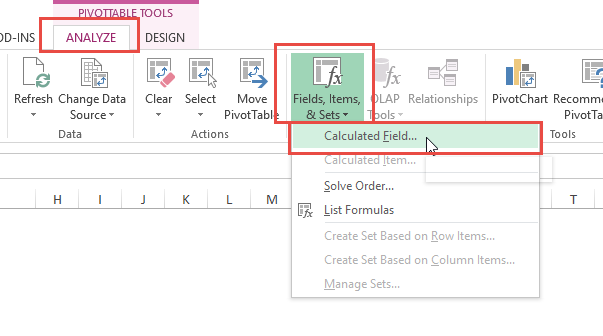Then from the Calculated Field dialog box give your field a name and type in the formula.  You can insert other Pivot Table fields from the fields area: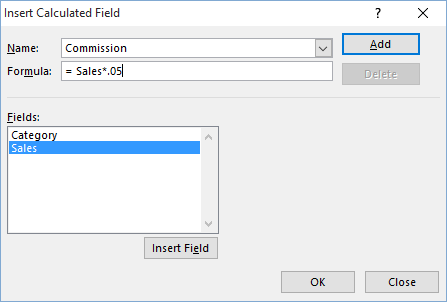When you click on the OK button, your new Calculated Pivot Field will be displayed as you see here: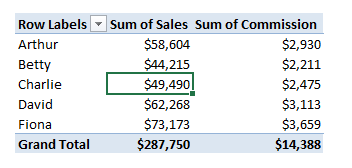2) Add a Calculated Field on a Calculated Field

You can also add Calculated Fields that utilize other Calculated Fields in your Pivot Tables.

Follow the same process as before but choose your newly created fields in your calculations as you see here: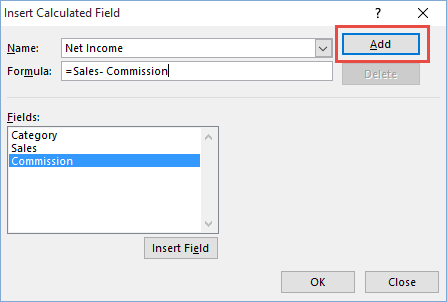You new Pivot Table field will be shown as you see here: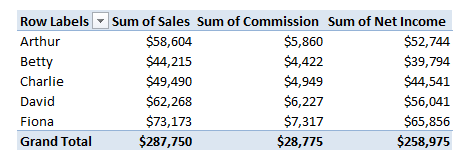3) Modify a Pivot Table Calculated Field

If you make a mistake or need to modify your calculated fields you can do that as well, but it is not as intuitive.  As before, you must first click on your Pivot Table and then the Fields button, however, the next step is not very intuitive.  To modify the calculated field, first select it from the Name drop down list instead of the Fields area.  Then you can modify the Formula bar and finally you can click on the Modify button as you see here: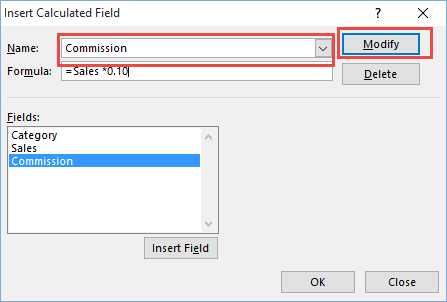4) Delete an Excel Calculated Field from a Pivot Table

Similarly to the modification process, to Delete a Calculated field, you must first select the field from the Name drop down box instead of the Fields area.  Then you can delete the field with the Delete button as you see here: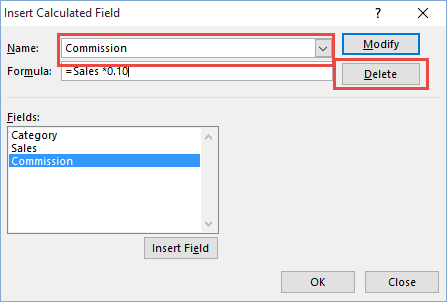Video Demonstration:

Check out this quick video tutorial on how to add a calculated field to your Excel Pivot Table:

Here is a sample file for you to try the Pivot Table Calculated Field processes:

What other options do you use with calculated fields in Excel Pivot Tables?  Let me know in the comments below.

Steve=True

Want to Receive the Next Post?